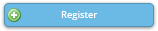Legend:
CC = Baltimore Convention Center,    H = Hilton Baltimore
* = applied session       ! = JSM meeting theme

#### Activity DetailsTweet CE_09C Sun, 7/30/2017, 8:30 AM - 5:00 PM H-Key Ballroom 8 Regression Modeling Strategies (ADDED FEE) — Professional Development Continuing Education Course ASA , Biometrics Section All standard regression models have assumptions that must be verified for the model to have power to test hypotheses and for it to be able to predict accurately. Of the principal assumptions (linearity, ad­ditivity, distributional), this course will emphasize methods for assessing and satisfying the first two. Practical but powerful tools are presented for validating model assumptions and presenting model results. This course provides methods for estimating the shape of the relationship between pre­dictors and response using the widely applicable method of augmenting the design matrix using restricted cubic splines. Even when assumptions are satisfied, over-fitting can ruin a model's predictive ability for future ob­servations. Methods for data reduction will be introduced to deal with the common case where the number of potential predictors is large in com­parison with the number of observations. Methods of model validation (bootstrap and cross-validation) will be covered, as will auxiliary topics such as modeling interaction surfaces, variable selection, overly influential observations, collinearity, and shrinkage, and a brief introduction to the R rms package for handling these problems. The methods covered will apply to almost any regression model, including ordinary least squares, logis­tic regression models, ordinal regression, quantile regression, longitudinal data analysis, and survival models. Instructor(s): Frank Harrell, Vanderbilt University